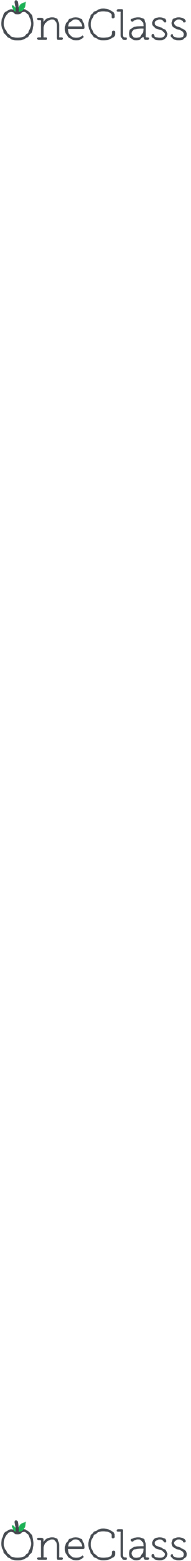Textbook Notes (270,000)
CA (160,000)
York (10,000)
INTL (3)
Chapter 2

# INTL 2200 Chapter Notes - Chapter 2: Transaction Cost, Absolute Advantage, Autarky

Department
International
Course Code
INTL 2200
Professor
Mokhles Hossain
Chapter
2

Page:
of 5CH2, Ricardian Model
unit-labour cost
(labour compensation / labour productivity ) adjusted by exchange rate
ER is function of interest rate differentiation
current account: goods and services primarily. Investment Income is not an investment
transaction, it is the income generated from investment.
capital/investment/financial accounts: asset transaction
Classical (Ricardian) Trade Theory:
Comparative Advantage -> Based on its productivity/technology difference
-2 Nations: Home (H) and Foreign (F)
-2 Goods/Commodities: Agriculture as in a bushel of wheat, (x) and Manufacture,
as in a meter of cloth, (y)
-1 input: Labour (L)
-x = f(L), y = f(L)
-“Workers make machines, machines aren’t independent entities, workers make
them work”
-Ricardo assumes labour (L) is homogeneous, that productivity is constant,
therefore:
-x = productivity of x * Lx
-y = productivity of y * Ly
-x = ax *Lx ax,ay = productivity measures
-y = ay *Ly
-ax = x / Lx
-ay = y / Ly HOME
-x = ax* * Lx*
-y = ay* * Ly* FOREIGN
-Lx + Ly = Lbar, size of the labour force
-Lx* +Ly* = Lbar*
Now suppose:
-ax = 1/2, ay = 1/5
-ax* = 1/20, ay* = 1/10
-from ax and ay, 2L = 1x and 5L = 1y
-from ax* and ay*, 20L* = 1x and 10L* = 1y
-L is the labour requirement per unit of output.
find more resources at oneclass.com
find more resources at oneclass.com
CH2, Ricardian Model
X (agri) Y (manu)
Home 2L 5L
Foreign 20L 10L
-Home has absolute advantage in both, but comparative in X, and foreign has
absolute DISadvantage in both, but comparative in Y.
Therefore:
Home exports X (imports Y)
Foreign exports Y (imports X)
COST TABLE X Y
Home 2/5Y 5/2X 1x = 2/5y
Foreign 2Y 1/2X 1x = 2Y
Comparative Adv.
Autarky:
Pre-trade Relative Price Pre-trade Relative Price
(Px/Py)Home = 2/5 < Range of Terms of Trade < (Px/Py)Foreign = 2
say:
Px/Py world = 1
then: 1x = 1y
then: 2/5x = 2/5y
Each time it exports X, it gains 3/5y
Production Possibility Frontier (PPF) : all possible combinations that each country can
produce individually.
HOME:
Max y: Lbar/5 = Lbarx * ay
Max x: Lbar/2 = Lbarx * ax
FOREIGN:
Max y: Lbar*/10 = Lbar* * ay*
Max x: Lbar*/5 = Lbar* * ax*
find more resources at oneclass.com
find more resources at oneclass.com
CH2, Ricardian Model
Slope is a straight line on the graph, not a curve because Rise/Run, because we
assumed that there is no law of diminishing returns in the Ricardian Model, since there’s
only one factor of production, which is labour:
(Lbar/5)/ (Lbar/2) = 2/5
(Lbar*/10) / (Lbar*/20) = 2
Note: Same as relative prices
=MRT (Marginal rate of transformation)
= MCx / MCy = Px/Py
The slope represents the ratio to transform two different types of products (agriculture to
manufacturing, and vice versa)
=1/5 / 1/2 = ay / ax = MPL Ysector / MPL Xsector
MRT is the ratio of two marginal products, or two marginal costs
Ricardian Model is underscored by constant cost, and constant productivity, where
labour is homogenous and the only factor in production.
Value of output produced = GDP = (Px * Xprodlevel) + (Py * Yprodlevel) = Px *
Xconsumption + Py * Yconsumption
We spend everything since there’s no saving in this model, since saving is a financial
account.
So when a country is gonna only produce either X or Y, to specialize, they will move all
the way to the end of their PPF. This is called complete specialization.
In a post trade environment, each country will commit to complete specialization and
thus either produce all X and no Y, or all Y and no X. Then trade will happen. The
beauty of trade is that the trade frontier is beyond both countries respective production
find more resources at oneclass.com
find more resources at oneclass.com